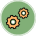•Production Linked Incentives Schemes in India

# COVID-19: Quantifying the infection risk of reopeningBy:If we open one train from Delhi, the probability that at least one person would be infected is 23%. This hyper-geometric probability is approximated as a binomial. It can be used to determine the number of people allowed to gather to keep this risk below a certain threshold.

1. Introduction
There are 12 bulbs in a box. Three of those are defective. An inspector draws a sample of five bulbs. What is the probability that at least one of them is defective? This is a model representation of the problem we face in calculating the probability of at least one infected person in a sample of 2000(Number of people in a train ) in a city with a population of 1.9 crore and 2,500 infected people. Since the known infected people are under quarantine, this study assumes an equal number of undetected patients. This could be because they are asymptomatic or have willfully not disclosed their condition.

2. Method

2.1 Basics

Population of at least one infected person is

1 − PNoinfectedperson = 1 − P2000non−infectedpeople

2.2 Estimating non-infected probability

The probability of one person being infection free isProbability that the second person is also infection free is:= (0.99986842105)
= 0.99973685941

Similarly probability that 2000 people are infection free is2.3 Probability of an infected person

1- 0.7686072184 = 0.23 = 23%

Therefore, there is a 23% chance that at least one person out of the 2000 is infected.

3. Numbers for select cities

The chance that there is at least one COVID-19 infected patient if one train is started:

Train in Delhi: 23%
Train in Mumbai: 35% Train in Chennai: 10%
Train in Pune: 46%